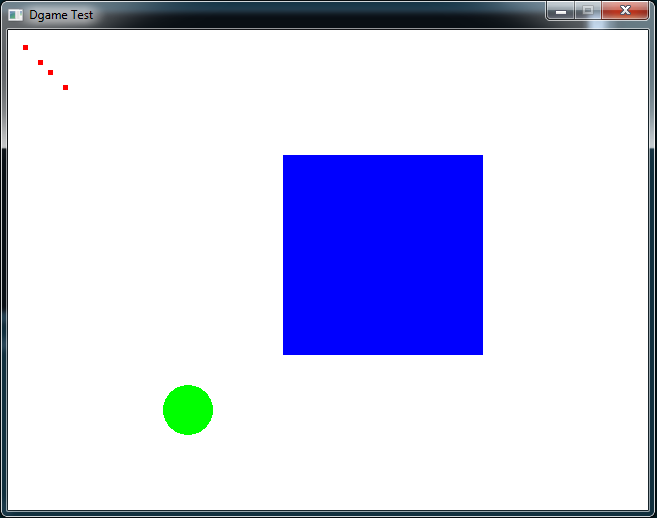# Draw Shapes

Let's draw Shapes. Dgame offers the Shape class with many many options. Let's get to know a few of them.

In this example we will create three Shapes: A big blue Quad, a Circle and a few smaller Quads.

To create Shapes you specify which geometry you want to have and set the coordinates for each Vertex. Of course you can do this (or change it) even after you've called the CTor. The Shape can be moved, transformed, scaled, colorized and filled etc. It also can change its color and geometry.

Let's try the geometry change: If you press the T key, the application checks if our Quad is still a Quad. If, we change the geometry to `Geometry.Triangles` to get a triangle, otherwise we change it back to a `Geometry.Quads`. And if we press the space key we move our circle to the right upper corner.

You should know, that the setPosition method, which Shape inherits from Transformable, has a slightly different effect on Shape as on e.g. Sprite, because Shape has already vertices which holds positions. So the position you specify is "added" to the vertex positions.

As always we have to draw each Shape object with a call to `wnd.draw(...)`

Show Raw
```import std.stdio;

import Dgame.Window.Window;
import Dgame.Window.Event;
import Dgame.Graphic;
import Dgame.Math;
import Dgame.System.Keyboard;

void main() {
Window wnd = Window(640, 480, "Dgame Test");

[
Vertex( 75,  75),
Vertex(275,  75),
Vertex(275, 275),
Vertex( 75, 275)
]
);

qs.fill = Shape.Fill.Full;
qs.setColor(Color4b.Blue);
qs.setPosition(200, 50);

Shape circle = new Shape(25, Vector2f(180, 380));
circle.setColor(Color4b.Green);

[
Vertex(55, 55),
Vertex(60, 55),
Vertex(60, 60),
Vertex(55, 60),
Vertex(15, 15),
Vertex(20, 15),
Vertex(20, 20),
Vertex(15, 20),
Vertex(30, 30),
Vertex(35, 30),
Vertex(35, 35),
Vertex(30, 35),
Vertex(40, 40),
Vertex(45, 40),
Vertex(45, 45),
Vertex(40, 45)
]
);
many.fill = Shape.Fill.Full;
many.setColor(Color4b.Red);

bool running = true;

Event event;
while (running) {
wnd.clear();

while (wnd.poll(&event)) {
switch (event.type) {
case Event.Type.Quit:
writeln("Quit Event");

running = false;
break;

case Event.Type.KeyDown:
if (event.keyboard.key == Keyboard.Key.T) {
qs.geometry = Geometry.Triangles;
else
} else if (event.keyboard.key == Keyboard.Key.Space)
circle.move(4, -4);
break;

default: break;
}
}

wnd.draw(qs);
wnd.draw(many);
wnd.draw(circle);

wnd.display();
}
}```

You should see this: